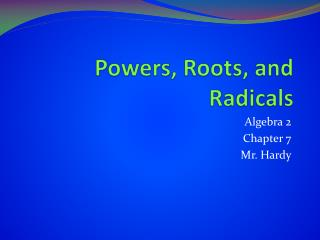DownloadDownload PresentationTélécharger la présentation- - - - - - - - - - - - - - - - - - - - - - - - - - - E N D - - - - - - - - - - - - - - - - - - - - - - - - - - -
##### Presentation Transcript

1. Powers, Roots, and Radicals Algebra 2 Chapter 7 Mr. Hardy

2. DO NOW • Evaluate the expression • 1) √9 • 2) -√121 • 3) (√25)2 • Solve each equation • 4) x2 = 49 • 5) (x – 1)2 = 64

3. Chapter 7 Overview • Powers, roots, and radicals • How to use rational exponents and nth roots of numbers • How to perform operations with and find inverses of functions • How to graph radical functions and solve radical equations

4. Chapter 7.1 Lesson Opener • You will havetwominutes to complete the lesson opener in your notebook. • Evaluate • (2)(2)(2)(2) • (-2)4 • (-2)(-2)(-2) • (2)(2)(2)

5. nth roots • For an integer n greater than 1, if bn = a, then b is an nth root of a. • An nth root of a is written: • where nis the index of the radical

6. DO NOW • Page 400 • #1 – 13

7. Notice • An nth root can also be written as a power of a. • Suppose:

8. THEREFORE In General Where n is greater than one (positive)

9. Real nth Roots

10. Finding nth Roots • Find the indicated real nth root(s) of a • n = 2, a = 16 • n is even, and a > 0; therefore, a has two real nth roots • √16 • √16 = ±4

11. Example 2 • n = 3, a = -1 • n is odd; therefore, a has one real nth root • Hint: What to the power of 3 is -1?

12. Example 3 • n = 4, a = -16 • n is even, but a is negative. How many real nth roots?

13. Try These • n = 3, a = 125 • n = 3, a = 0 • n = 2, a = 49 • 5 • 0 • 7

14. Rational Exponents • Keep in mind: Rational exponents do not have to be in the form of 1/n. Other rational numbers can be used as exponents as well!

15. Example 1

16. Example 2

17. Try These • 9 • 7 • 1/27

18. Solving Equations • Example 1 • - 5x2 = -30 • x2 = 6 • x = ±√6 • Use a calculator to round the result • x ≈ ±2.45

19. Example 2 • (x + 4)3 = 27 • x + 4 = 3 (Take the cube root of both sides) • x = -1

20. Try These • x4 = 87 • 2x3 = 92 • (x – 1)5 = 12 • ±3.05 • 3.58 • 2.64

21. Your Turn Classwork Homework • Complete Practice B worksheet • Chapter 7.1, page 404 #14-60 even, 65-66 all

22. Challenge • Rationalize each denominator, and express the fraction in simplest form

23. Do Now 3-15-13 • Homework Quiz • Chapter 6.8 • #24, 26, 28 • Chapter 7.1 • #16, 28, 40, 56

24. Recall • Rewrite the Expression • 1) • 2) • 3) • Solve the equation. Round if necessary • 2x4 = 35 • 5x3 + 10 = 961

25. Section 7.2 Opener • Evaluate the expression • 1) 42 43 • 2) (22)3 • 3) 34/32 • 4) 2-3 • 5) (2  3)4

26. Properties of (Rational) Exponents

27. Product and Quotient Property

28. Using Properties of Rational Exponents • Example 1 • Same bases- ADD the EXPONENTS

29. Example 2 • MULTIPLY the EXPONENTS • (21)(x2) = 2x2

30. Example 3

31. Try These • x1/2 • 256 • y1/3 • 4

32. Simplest Form • For a radical to be in simplest form, you must apply the properties of radicals, remove any perfect nth powers (other than 1) and rationalize any denominators. • Two radical expressions are like radicals if they have the same index and the same radicand.

33. Examples • √2 * √8 • = √2*8 • =√16 • =4

34. Try These

35. Writing Radicals and Variable Expression in Simplest Form • Example 1 • Factor out the perfect fourth root • Use the Product Property

36. Example 2 • Make the denominator a perfect cube (in order to rationalize

37. Do Now Looseleaf Silently Agree or Disagree with the statement that follows and support it with specific and accurate facts that include explanations/proofs, sentences, number statements, and worked out mathematical solutions. The expression can be rewritten as

38. Recall • Simplify

39. Recall: nth roots w/ variables  RECALL from Chapter 5 FACTOR out the PERFECT SQUARE

40. Example 2

41. Try These

42. Application Use what you know to find a radical expression for the perimeter of the triangle. Simplify the expression. 4ft 1ft 2ft 2ft

43. The function f(x) = 70x3/4… • …models the number of calories per day, f(x), a person needs to maintain life in terms of that person's weight, x, in kilograms. (1 kilogram is approximately 2.2 pounds.) Use this model and a calculator to solve.Round answers to the nearest calorie. • How many calories per day does a person who weighs 80 kilograms (approximately 176 pounds) need to maintain life?70 kilograms (approximately 154 pounds)?